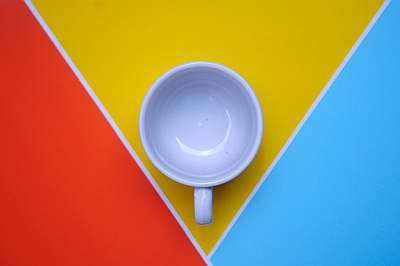# Liters to Ounces

Instructions: Use this calculator to convert liters to ounces, showing all the steps. Please type the number of liters you would like to convert in the form below:Type the number of liters:

## Using this Liters to Ounces Conversion Tool

This conversion tool will allow you to convert liters into ounces, a process that could make a lot of sense when dealing with volume measurement from other countries.

Naturally people are accustomed to their own volume units, and perhaps they are not aware that other countries may use some different and completely unfamiliar volume units. Then it is type of conversion tool will come in handy.

Liters and ounces are fairly commonly used volume units, which are broadly used, but not many people will know how many ounces are there in a liters, for example.## What is the equivalence of 1 liter in ounces?

This initial equivalence will aid us for the more general conversion formula. Indeed, the basic equivalence is that 1 liter is the same as 33.814 ounces.

This is the starting point to finding a general conversion tool, as we will see in the next section.

## How do you convert liters to ounces

In order to convert liters to ounces, you will need to multiply a constant by the number of liters, in order to get the equivalent number of ounces.

## What are the steps for the conversion of liters into ounces

• Step 1: Observe the basic equivalence: 1 liter = 33.814 ounces
• Step 2: Next, you need to identify that number of liters L you need to convert to fluid ounces
• Step 3: Now, we need to use the following direct proportion, based on Step 1: $$\displaystyle \frac{1}{33.814} = \frac{L}{O}$$
• Step 4: We solve for O, and we get the following conversion formula to convert liters to ounces: $$O = 33.814 L$$

## Why would you want to convert liters to ounces

Liter is the main volume unit in the metric system, whereas ounces is one of the most commonly used volume unit in the imperial system. That is why it is important to do the conversion.

Often times, especially when visiting other countries, you will likely find the need to doing this kind of conversions often.### Examples: Converting liters to fluid oz

Convert 1/2 liter to ounces

Solution: We have the following conversion formula:

$O = 33.814 L$

Now, we know we need to convert L = 1/2 liters, so we plug that into the formula:

$O = 33.814 L = 33.814 \times 1/2 = 16.907 \text{ oz}$

### Example: Liter ounces conversion

Convert 1.75 liters to ounces

Solution: Same as the example above, we use the formula:

$O = 33.814 L$

In this case, what we have is L = 1.75 liters, so now we plug that into the formula:

$O = 33.814 L = 33.814 \times 1.75 = 59.175 \text{ oz}$

### Examples: Another liters to ounces conversion

What is 3 oz to liters

Solution The conversion formula is:

$O = 33.814 L$

but in this case we know O, and not L. So we solve for L:

$O = 33.814 L \Rightarrow L = \displaystyle \frac{O}{33.814}$

So now, plugging O = 3 in, we get:

$L = \displaystyle \frac{O}{33.814} = \displaystyle \frac{3}{33.814} = 0.0887 \text{ liters}$

## Other useful volume unit conversions

Among the most common volume conversions are ounces to cups and cups to ounces, which derive their conversion formulas using direct proportion too.

Then, you may be interested in the inverse process of what we just did, which is going from ounces to liters.

Plus, you can make conversions within the imperial unit system, including cups to quarts, quarts to gallons and many others.